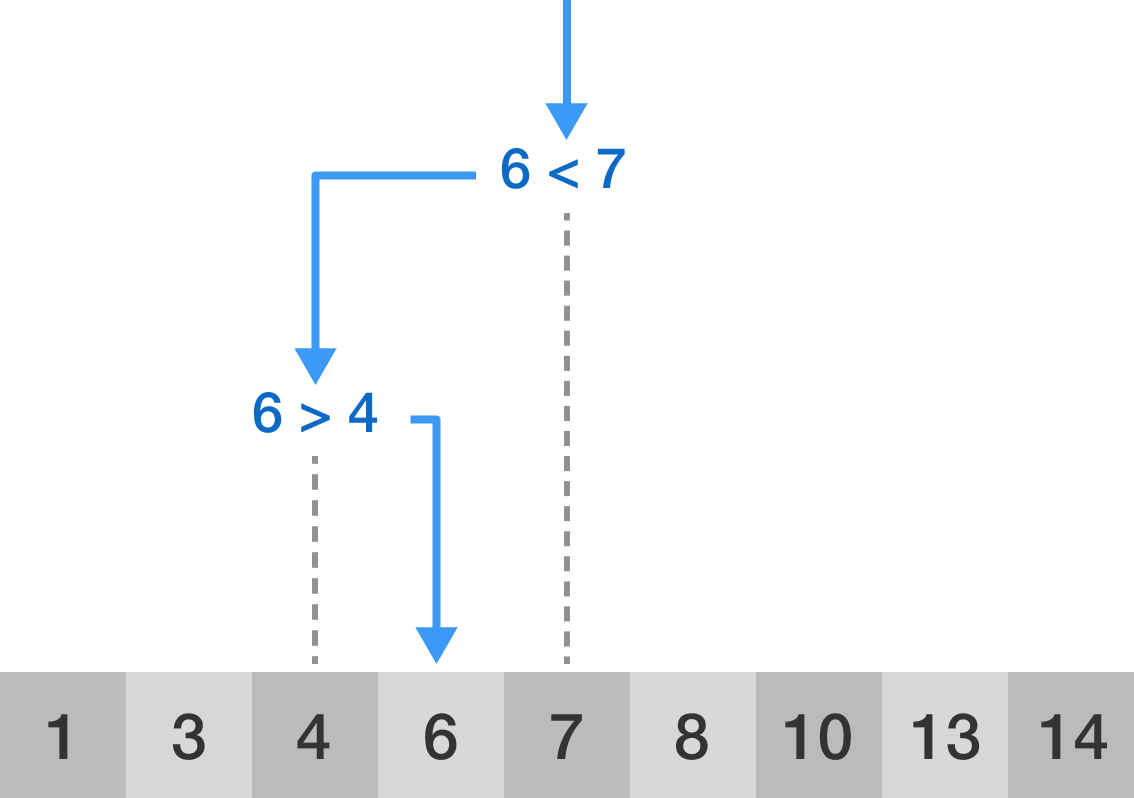### Data Structures

One of the simplest questions one might have about a list is whether or not some item is in the list. For example, if you are writing an algorithm to determine if someone should have access to a members-only website, you can solicit their information and then see if it matches some item in the members list.

Suppose that you have an unsorted array of 1000 emails and you want to check if abc123@gmail.com is in the array. Using a naive algorithm where you move sequentially through the list checking if each element matches abc123@gmail.com, what is the maximum number of comparisons between emails you would ever need to make?

Remember, it is entirely possible that the email is not in the array at all.

# Searching

The search algorithm described in the previous question is known as linear search. Clearly, the worst case is very bad, since you might have to go through every element in the array.

What is the best case for the number of comparisons you will need to make when searching for a specific email in an array of 1000 emails?

# Searching

So, for linear search, the best case is great (just 1 comparison!) but the worst case is very bad--possibly having to compare the email with every element in the array.

What about on average for this array of length 1000? If the email is in the array (whose elements are distinct and randomly arranged), what is the average number of comparisons that you will need to make?

(Note: While we are assuming the email is in the array, the algorithm can't make that assumption; it needs to confirm the presence of the email before it stops.)

# Searching

To summarize, to determine if an element is in an array with $n$ elements using linear search, the number of comparisons we need to make is

• in the best case, just 1 comparison;
• in the worst case, $n$ comparisons;
• in the average case, given that the element is actually in the array, $\frac{n+1}{2}$ comparisons.While the average case might seem not too bad, it’s important to think about what this means for large $n.$ While 500,000 comparisons is certainly not as bad as 1,000,000, they’re both problematic in that they scale linearly with $n;$ that is, with twice as much data, the algorithm will need twice as many comparisons.

This isn’t great in a world with increasingly large data sets. Can we do better?

# Searching

At first glance, it feels hard to do better than linear search. If—as with an unsorted list—we know nothing about the elements and their organization within the array, then we can’t do any better than just checking all of the elements.

But what if the list is sorted?

Define a comparison as an operation which takes in one number and tells you whether it is larger than, smaller than, or equal to, another.

Suppose you are given a sorted array with 1000 elements, and you can use at most $n$ comparisons to determine whether a certain number is in this array.

What is the smallest value of $n$ such that you will always be capable of making this determination, regardless of the values of the elements in the array?

Hint: Pick an element from the list to observe first. If the element we observe is greater than our target what does this tell us? What if it's lower? With a sorted list, knowing if an element is greater or less than the element we’re looking for can be useful!

# Searching

The search described in the previous problem is called binary search.Visualization of the binary search algorithm where 6 is the target value.

Consider a sorted array. In short, binary search repeatedly chooses a number in the middle of the remaining possible numbers, and then determines if the desired number would lie to the left or right of this chosen number (or exactly the chosen number). In each iteration, the amount of remaining numbers is halved, making binary search very efficient. This is especially important when dealing with a very large array.

# Searching

How much does the faster binary search matter? The short answer: a lot. Comparing linear search and binary search serves as a clear demonstration that the choice of algorithm matters.

Suppose you have a sorted array with 100,000,000 elements in it. Assuming the worst case for each method, what is the ratio between the number of comparisons made by linear search and the number of comparisons made by binary search?

In other words, approximately how many times could we run binary searches before making the number of comparisons required for a single linear search?

# Searching

Modern data sets tend to be massive; it’s critical to store and structure data in a way that makes common questions easily answerable. Throughout this course, we’ll consider what data types and structures should be used in various situations.

So far, we’ve already seen one repeated theme: lists tend to be more useful when sorted. But we haven’t answered the question of how to sort a list, and how computationally complicated it would be to do so. We’ll start to answer this question in the next quiz!

×